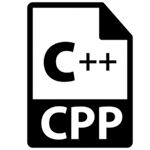# C++接口

• 抽象类
• 接口

## C++抽象类

```#include <iostream>
using namespace std;
class Shape
{
public:
virtual void draw()=0;
};
class Rectangle : Shape
{
public:
void draw()
{
cout < <"drawing rectangle..." < <endl;
}
};
class Circle : Shape
{
public:
void draw()
{
cout <<"drawing circle..." < <endl;
}
};
int main( ) {
Rectangle rec;
Circle cir;
rec.draw();
cir.draw();
return 0;
}```

```drawing rectangle...
drawing circle...```